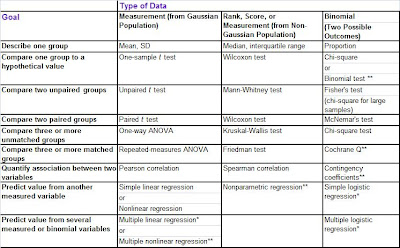# Use of Statistics – Practical Considerations

It is often confusing to decide on which statistic to use at what point. Also researchers need to be careful that the statistics they present does truly apply in the context of the problem. Statictics can be misleading and probably incorrect if used outside the boundaries set by its assumptions. In this post we analyse different statistics and what care should be taken while using them.

Mean,Median and mode : The mean is probably the most widely used number. A lot of claims are made using the mean but this could be quite misleading. The mean does not include any measure of variability. Also, outliers may distort the mean to a large extent. Median can be reported with the mean to get an idea of how the extreme looks like. For a more detailed analysis the box and whisker plot gives a fair idea of how the data looks like. It is therefore better to provide a measure of variablility along with the measure of central tendency.

Discrete distribution : While using binomail distribution the independence and size assumptions should be met when sampling is done without replacement. In Poisson distribution the size and lambda assumptions should be met. for binomial distributions for large sizes of n the probability at a particular x value should be reported with care. for example in a coin toss with n = 100 and p=0.5 P(x=50)=0.076. This could be counter-intuitive and hence a better way to report this is to use P(x>50). In poisson study the value of lambda may change and hence the researcher should make sure that lambda is valid for their test conditions.

Continuous distribution : Here again, the value of lambda should be chosed with care. The value of lambda used in one study may not be useful in other similar study since the populations may be different or the time interval of the lambda may be different. For normal distribution, care should be taken to verify the distribution since most of the tests are hightly sensitive to the type of distribution of the population.

Sampling – One of the widely misused method is sampling. Many surveys use non random sampling instead of random sampling and the statistic thus obtained may quite off the mark. The sampling data is quite often used to make inferences about the population and if questionable means have been used to sample data then the population inferences may be highly incorrect.

Hypothesis testing for single population: In hypothesis testing it is imperative that the researcher formulate the hypothesis in such a way that what is known is the null hypothesis and what he strives to prove is the alternate hypothesis. Researchers may use null hypothesis as the statement of what they want to prove and this is incorrect since then the theory is assumed to be true and alternate hypothesis only strives to disprove it. While using t-test, the population needs to be normally distributed to some extent. However, the chi square test is extremely sensitive to the assumption that the population is normally distributed and hence the researcher should make sure that the population is indeed normally distributed. Also, the business implication of a statistical significance test needs to be worded carefully. The context needs to be understand and the assumptions while arriving at the ‘significance’ level should be clarified.

Hypothesis testing for two populations : The assumptions used while using the statistic that compares two populations should be met. 1) For small sample sizes, the x test is valid only if the population is normally distributed and population variances are known. 2) t-test can be used if population is normally distributed and population variances are assumed to be equal.3) For F test the two populations should be normally distribued.

ANOVA: While reporting ANOVA results the researcher needs to consider all variables that may effect the outcome of the experiment. She should at least mention the concomitant variables that have not been considered in the experiment but that have been shown to show some influence to the dependent variable, however small that dependence may be. The treatment levels selected for the study should possibly be random. Certain tests such as two way factorial design or completely randomized design with Tukey’s HHSD may require equal smaple sizes. Sometimes researcher arbitrarily make up or delete values to make sample sizes equal and this is incorrect.

Regression Analysis: regression analysis require equal error variance and independence of error terms. Residual and other statistical techniques can be used to verify that. Remember that the regression line is valid only if the assumptions are met. Another problem arises when the regression model is used outside the values used to formulate the regression model. The model is valid only in the domain used to create the model. Data may behave linearly in a certain range but may tend to behave non linearly outside this range.

Multiple Regression: For a small degree of freedom the value of R squared obtained may be inflated. A cause and effect relationship may be assumed to occur between the dependent variable and the predictors. It is however possible that factors not considered in the study may be causing the behaviour. Also for multiple variables with different units the R squared values should not be compared. The coefficients of regression should also not be used to compare the effect of various predictors since the predictors may have different units. Also while building the multiple Regression model it is not necessary that the variables that enters the equation first is the most influential.

Reference;

Use the site http://www.whichtest.info/ to figure out which test to use when.

Also a table is available at http://www.graphpad.com/www/Book/Choose.htm that assists in understanding which test to use when. The table is reproduced below.